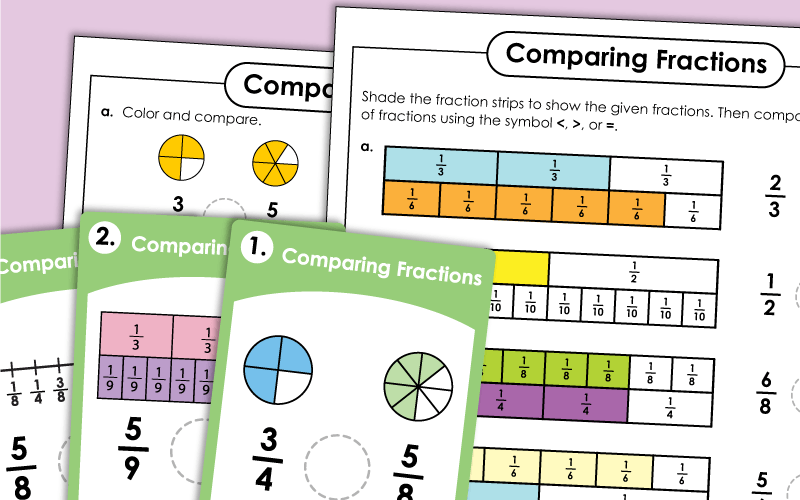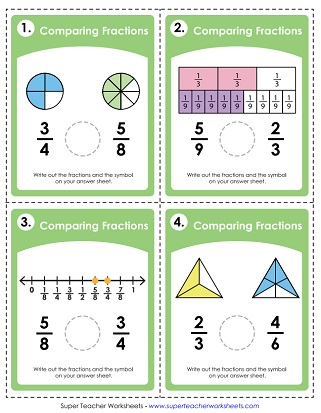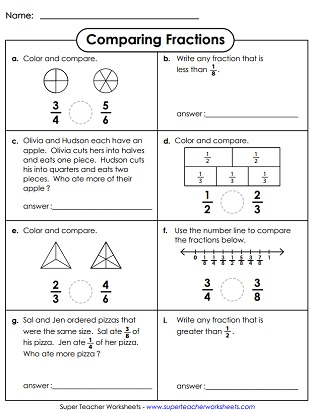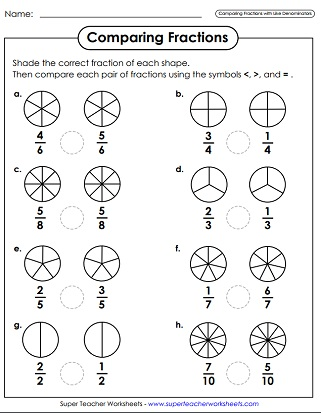# Comparing and Ordering Fractions

Compare and order fractions and mixed numbers. Includes "greater than," "less than," and "equal to" symbols. This page has printable task cards, learning center games, and worksheets.## Comparing Fractions

On this printable worksheet, student will compare fractions using a variety of methods, including shape illustrations, fraction strips, and number lines.
Shade the fraction strips (tape diagrams) to show the given fractions. Then compare, using the mathematical symbols <, >, or =.
Compare pairs of fractions with like denominators. (example: four-sixths is less than five-sixths)
Compare pairs of fractions with different denominators. Each fraction has an illustration to help. (example: one-third is greater than one-fourth)
First, find each fraction on the number line. Compare the fractions using the less than, greater than, or equal to symbols. Students can use the number line to help.
In each word problem, students will compare a pair of fraction numbers and determine which one has the greater value.
Use mathematical notation (symbols for greater than, equal to, less than) to compare the fractions.
First, students color the pairs of fraction circles according to the directions. Then they will determine which one of the two fractions is greater.
This file has 30 task cards on comparing fractions. Each one has a model/illustration to help.
Use the mathematical symbols <, >, and = to compare each pair of fractions. This set includes 30 task cards. Great for small group instruction, class games, peer study groups, or learning centers.
This file has a set of word, phrase, and number cards. Use them for teaching students to compare fractions in small group settings, learning centers, or classroom games.

## Comparing Mixed Numbers

Pupils will find the value of each mixed number on the number line. Then use the <, >, and = symbols to compare pairs of mixed numbers.

## Comparing Measurements

Compare linear measurements. Units include miles, yards, feet, and inches. (example: 3/4 of a yard > 25 inches)

## Fraction Counting

Count by halves.  Great for helping students learn to measure inches on a ruler.
Count by fourths.  Excellent for helping students learn to measure to the nearest quarter inch on a ruler.

## Ordering Fractions

Cut out the fraction cards and lay them on the table. Then arrange the fraction cards on the table from least to greatest. This activity works well as a learning center or small group activity.
The dancing pigs have fractions on them.  Cut out the pigs and place them in order from least to greatest.

## Ordering Mixed Numbers

Cut out the mixed number game cards. Lay them out in a row, from least to greatest. Use this activity for cooperative learning activities, independent practice, or as a learning center.

## Reference Chart

This chart shows order of fractions, equivalent fractions, and simplest form. Use for comparing, ordering, and simplifying common fractions with denominators up to 12.
Fraction Worksheets

Learn about equivalent fractions, simplifying fractions, and fractions of sets. There are also links to fraction addition, multiplication, subtraction, and division.

Mixed Numbers

Learn all about mixed numbers with these printable lesson activities.

Comparing Numbers

From this index page, you can jump to worksheets on comparing 4-digit numbers, 5-digit numbers, or 6-digit numbers. Also includes links to STW resources on comparing decimals and money.

Comparing Decimals

Here are some learning resources for teaching ordering and comparing of decimal numbers.

## Worksheet ImagesMy Account
Site Information